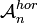# VS, TS and TG Algebras

(diff) ← Older revision | Latest revision (diff) | Newer revision → (diff)

## Abstract

We introduce VS-, TS- and TG-Algebras; three types of algebraic entities within which some basic equations of knot theory (related to Algebraic Knot Theory) can be written and potentially solved. Why?

• These equations are valuable yet not well understood. My hope is to study them in many simpler spaces (i.e., in many simpler VS-, TS- and TG-Algebras) than the ones that naturally occur in knot theory, in the hope that out of many test cases an understanding will emerge.
• In particular, one day I hope to write (or encourage the writing of) computer programs that will take a VS-, TS-, or TG-algebra "plug-in" and given it, will carry out all the necessary higher-level algebra. This will make it easier to study particular cases computationally. But for this, the notions of VS-, TS- and TG-Algebras must first be completely specified.

### VS-Algebras in One Paragraph

A VS-Algebra (Vertical Strands Algebra) is an algebraic object that is endowed with the same operations as the algebras${\mathcal A}_n^{hor}$ of horizontal chord chord diagrams - multiplication (vertical stacking), strand permutation, strand addition, strand doubling and strand removal. It is local if it satisfies the same "locality in time" and "locality in space" relations that${\mathcal A}_n^{hor}$ satisfies . In any local VS-algebra the equations for a Drinfel'd associator (i.e., the pentagon and the hexagon) can be written and potentially be solved, and solutions always lead to braid invariants. Likewise in any local VS-algebra the largely undocumented braidor equations can be written and potentially be solved, and solutions always lead to braid invariants.

A TS-Algebra